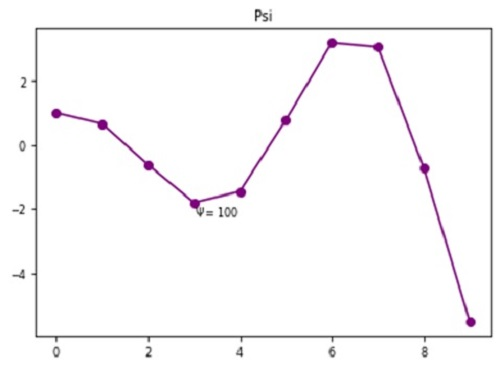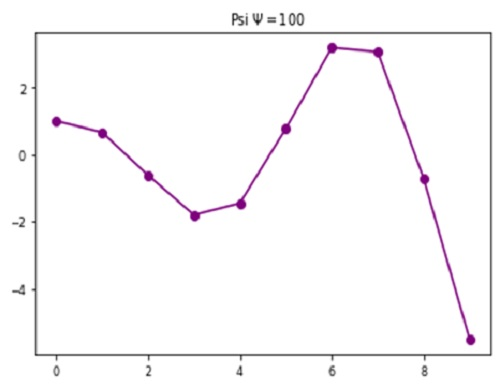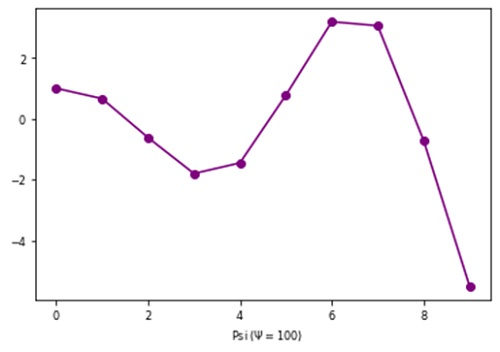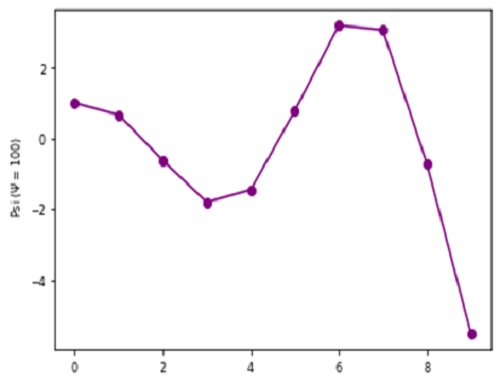# Python | Adding Psi in Plot Label

Here, we are going to learn how to add psi in plot label in Python?
Submitted by Anuj Singh, on August 07, 2020

Ψ (Psi) is very often used greek mathematical letters. They are mostly used in physics and other mathematical expressions, especially, when there is topic related change (for example charge and electrodynamics) and therefore, they have registered importance in languages.

Therefore, matplotlib has defined a command for usage.

```plt.text(3, 0.4, r'\$\Psi=100\$')
``````plt.title(‘Psi 'r'\$\Psi=100\$')
#Adding Ψ in title of the figure
``````plt.xlabel('Psi ('r'\$\Psi=100)\$')
#Adding Ψ in x axis label of the figure
``````plt.ylabel('Psi ('r'\$\Psi=100)\$')
#Adding Ψ in y axis label of the figure
```## Python code for adding psi in plot label

```import numpy as np
import matplotlib.pyplot as plt

x = np.arange(10)
y = np.cos(x)*np.exp(x/5)

#  Psi
# In text
plt.figure()
plt.plot(x,y, '-o', color=  'purple')
plt.title('Psi')
plt.text(3, -2.2, r'\$\Psi=100\$')
plt.show()

# In title
plt.figure()
plt.plot(x,y, '-o', color=  'purple')
plt.title('  Psi 'r'\$\Psi=100\$')
plt.show()

# In x-axis label
plt.figure()
plt.plot(x,y, '-o', color=  'purple')
plt.xlabel('  Psi ('r'\$\Psi=100)\$')
plt.show()

# In y-axis label
plt.figure()
plt.plot(x,y, '-o', color=  'purple')
plt.ylabel('  Psi ('r'\$\Psi=100)\$')
plt.show()
```

Output:

```Output is as Figure
```

Languages: » C » C++ » C++ STL » Java » Data Structure » C#.Net » Android » Kotlin » SQL
Web Technologies: » PHP » Python » JavaScript » CSS » Ajax » Node.js » Web programming/HTML
Solved programs: » C » C++ » DS » Java » C#
Aptitude que. & ans.: » C » C++ » Java » DBMS
Interview que. & ans.: » C » Embedded C » Java » SEO » HR
CS Subjects: » CS Basics » O.S. » Networks » DBMS » Embedded Systems » Cloud Computing
» Machine learning » CS Organizations » Linux » DOS
More: » Articles » Puzzles » News/Updates